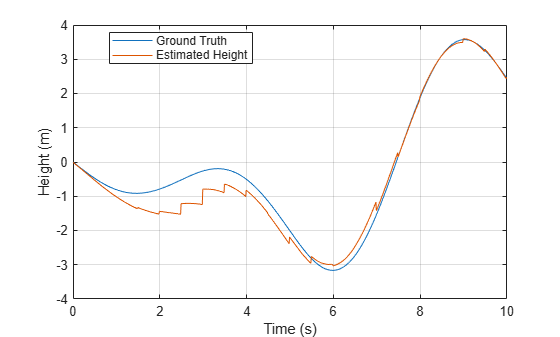# ahrs10filter

Height and orientation from MARG and altimeter readings

Since R2019a

## Description

The `ahrs10filter` object fuses MARG and altimeter sensor data to estimate device height and orientation. MARG (magnetic, angular rate, gravity) data is typically derived from magnetometer, gyroscope, and accelerometer sensors. The filter uses an 18-element state vector to track the orientation quaternion, vertical velocity, vertical position, MARG sensor biases, and geomagnetic vector. The `ahrs10filter` object uses an extended Kalman filter to estimate these quantities.

## Creation

### Syntax

``FUSE = ahrs10filter``
``FUSE = ahrs10filter('ReferenceFrame',RF)``
``FUSE = ahrs10filter(___,Name,Value)``

### Description

````FUSE = ahrs10filter` returns an extended Kalman filter object, `FUSE`, for sensor fusion of MARG and altimeter readings to estimate device height and orientation.```
````FUSE = ahrs10filter('ReferenceFrame',RF)` returns an extended Kalman filter object that estimates device height and orientation relative to the reference frame `RF`. Specify RF as `'NED'` (North-East-Down) or `'ENU'` (East-North-Up). The default value is `'NED'`. ```

example

````FUSE = ahrs10filter(___,Name,Value)` sets each property `Name` to the specified `Value`. Unspecified properties have default values.```

## Properties

expand all

Sample rate of the IMU in Hz, specified as a positive scalar.

Data Types: `single` | `double`

Multiplicative process noise variance from the gyroscope in (rad/s)2, specified as positive real finite numbers.

Data Types: `single` | `double`

Multiplicative process noise variance from the accelerometer in (m/s2)2, specified as positive real finite numbers.

Data Types: `single` | `double`

Multiplicative process noise variance from the gyroscope bias in (rad/s2)2, specified as positive real finite numbers.

Data Types: `single` | `double`

Multiplicative process noise variance from the accelerometer bias in (m/s2)2, specified as positive real finite numbers.

Data Types: `single` | `double`

Additive process noise for geomagnetic vector in μT2, specified as positive real finite numbers.

Data Types: `single` | `double`

Additive process noise for magnetometer bias in μT2, specified as positive real finite numbers.

Data Types: `single` | `double`

State vector of the extended Kalman filter. The state values represent:

StateUnitsIndex
Orientation (quaternion parts)N/A1:4
Altitude (NED or ENU)m5
Vertical Velocity (NED or ENU)m/s6
Delta Velocity Bias (XYZ)m/s10:12
Geomagnetic Field Vector (NED or ENU)μT13:15
Magnetometer Bias (XYZ)μT16:18

The default initial state corresponds to an object at rest located at `[0 0 0]` in geodetic LLA coordinates.

Data Types: `single` | `double`

State error covariance for the Kalman filter, specified as an 18-by-18-element matrix of real numbers.

Data Types: `single` | `double`

## Object Functions

 `predict` Update states using accelerometer and gyroscope data for `ahrs10filter` `fusemag` Correct states using magnetometer data for `ahrs10filter` `fusealtimeter` Correct states using altimeter data for `ahrs10filter` `correct` Correct states using direct state measurements for `ahrs10filter` `residual` Residuals and residual covariances from direct state measurements for `ahrs10filter` `residualmag` Residuals and residual covariance from magnetometer measurements for `ahrs10filter` `residualaltimeter` Residuals and residual covariance from altimeter measurements for `ahrs10filter` `pose` Current orientation and position estimate for `ahrs10filter` `reset` Reset internal states for `ahrs10filter` `stateinfo` Display state vector information for `ahrs10filter` `tune` Tune `ahrs10filter` parameters to reduce estimation error `copy` Create copy of `ahrs10filter`

## Examples

collapse all

Load logged sensor data, ground truth pose, and initial state and initial state covariance. Calculate the number of IMU samples per altimeter sample and the number of IMU samples per magnetometer sample.

```load('fuse10exampledata.mat', ... 'imuFs','accelData','gyroData', ... 'magnetometerFs','magData', ... 'altimeterFs','altData', ... 'expectedHeight','expectedOrient', ... 'initstate','initcov'); imuSamplesPerAlt = fix(imuFs/altimeterFs); imuSamplesPerMag = fix(imuFs/magnetometerFs);```

Create an AHRS filter that fuses MARG and altimeter readings to estimate height and orientation. Set the sampling rate and measurement noises of the sensors. The values were determined from datasheets and experimentation.

```filt = ahrs10filter('IMUSampleRate',imuFs, ... 'AccelerometerNoise',0.1, ... 'State',initstate, ... 'StateCovariance',initcov); Ralt = 0.24; Rmag = 0.9;```

Preallocate variables to log height and orientation.

```numIMUSamples = size(accelData,1); estHeight = zeros(numIMUSamples,1); estOrient = zeros(numIMUSamples,1,'quaternion');```

Fuse accelerometer, gyroscope, magnetometer and altimeter data. The outer loop predicts the filter forward at the fastest sample rate (the IMU sample rate).

```for ii = 1:numIMUSamples % Use predict to estimate the filter state based on the accelometer and % gyroscope data. predict(filt,accelData(ii,:),gyroData(ii,:)); % Magnetometer data is collected at a lower rate than IMU data. Fuse % magnetometer data at the lower rate. if ~mod(ii,imuSamplesPerMag) fusemag(filt,magData(ii,:),Rmag); end % Altimeter data is collected at a lower rate than IMU data. Fuse % altimeter data at the lower rate. if ~mod(ii, imuSamplesPerAlt) fusealtimeter(filt,altData(ii),Ralt); end % Log the current height and orientation estimate. [estHeight(ii),estOrient(ii)] = pose(filt); end```

Calculate the RMS errors between the known true height and orientation and the output from the AHRS filter.

```pErr = expectedHeight - estHeight; qErr = rad2deg(dist(expectedOrient,estOrient)); pRMS = sqrt(mean(pErr.^2)); qRMS = sqrt(mean(qErr.^2)); fprintf('Altitude RMS Error\n');```
```Altitude RMS Error ```
`fprintf('\t%.2f (meters)\n\n',pRMS);`
``` 0.38 (meters) ```

Visualize the true and estimated height over time.

```t = (0:(numIMUSamples-1))/imuFs; plot(t,expectedHeight);hold on plot(t,estHeight);hold off legend('Ground Truth','Estimated Height','location','best') ylabel('Height (m)') xlabel('Time (s)') grid on`````` fprintf('Quaternion Distance RMS Error\n');```
```Quaternion Distance RMS Error ```
`fprintf('\t%.2f (degrees)\n\n',qRMS);`
``` 2.93 (degrees) ```

## Version History

Introduced in R2019a